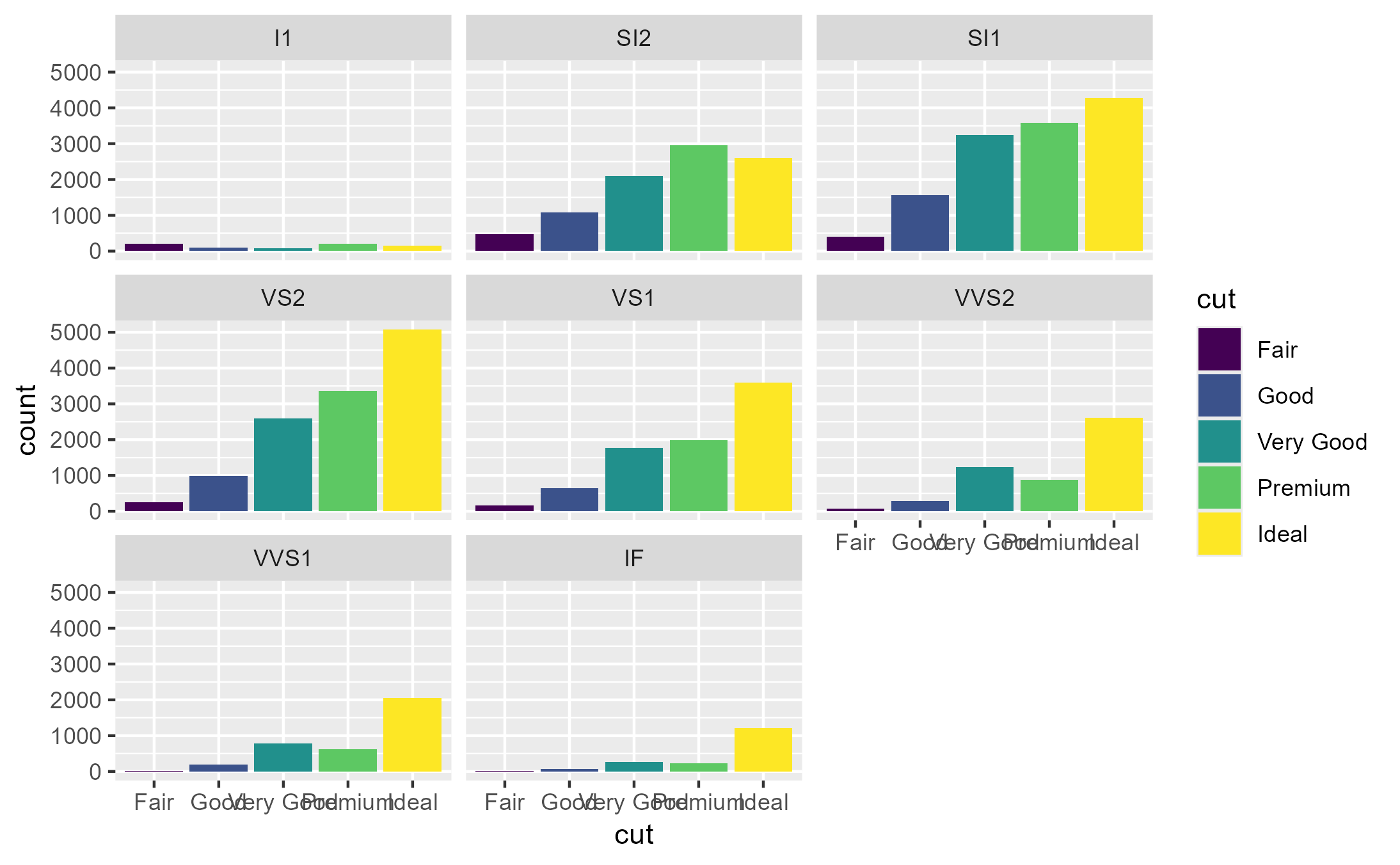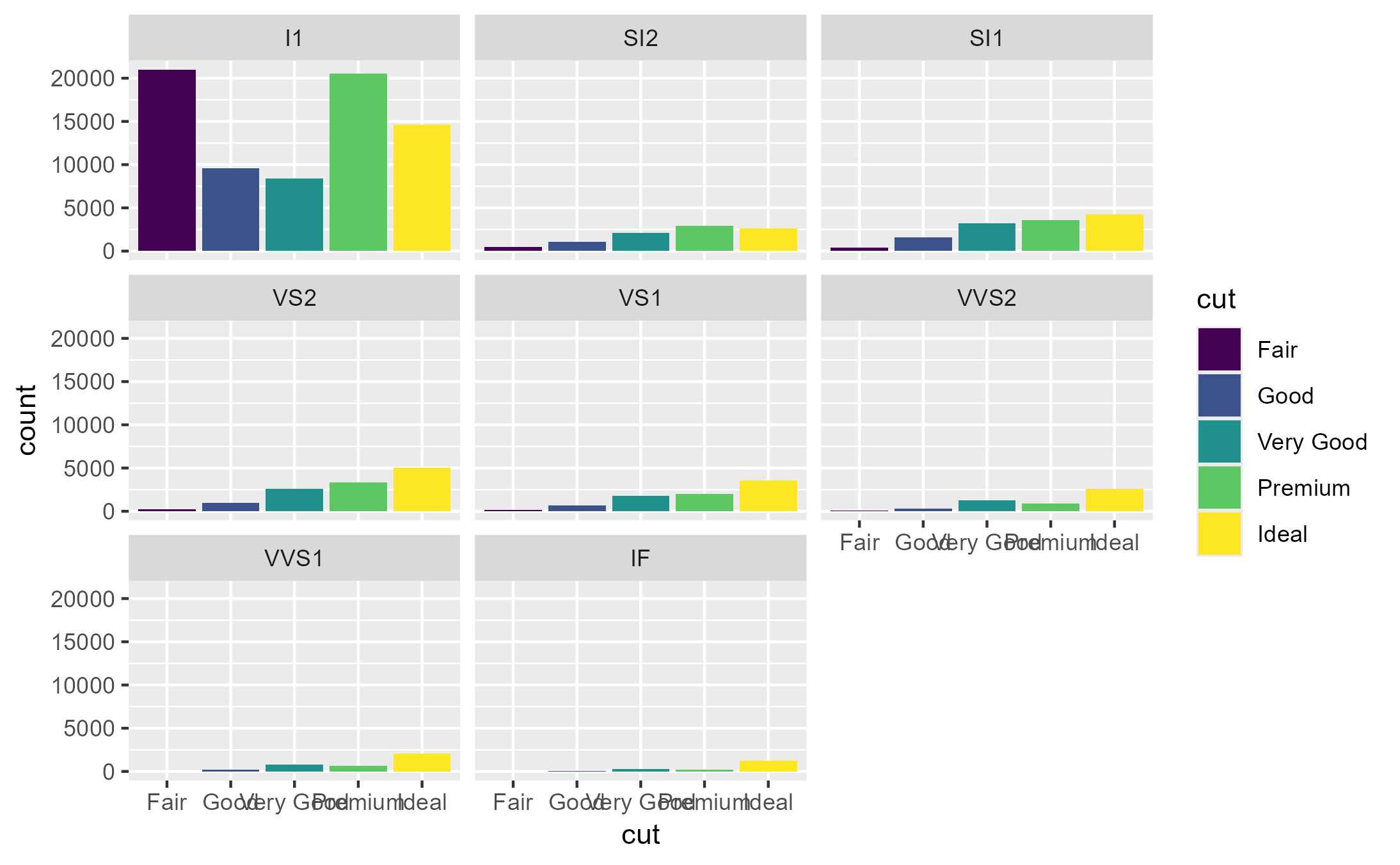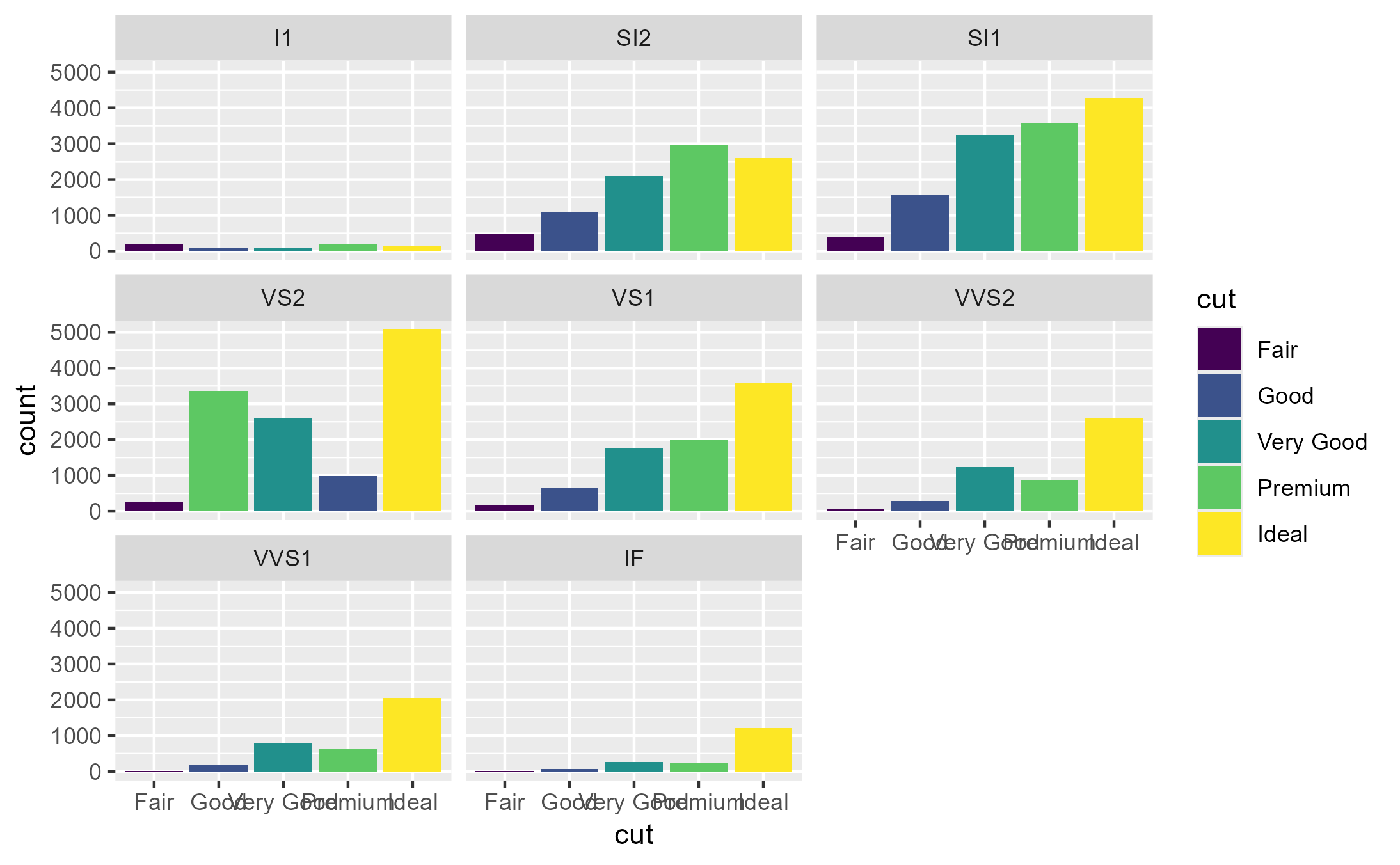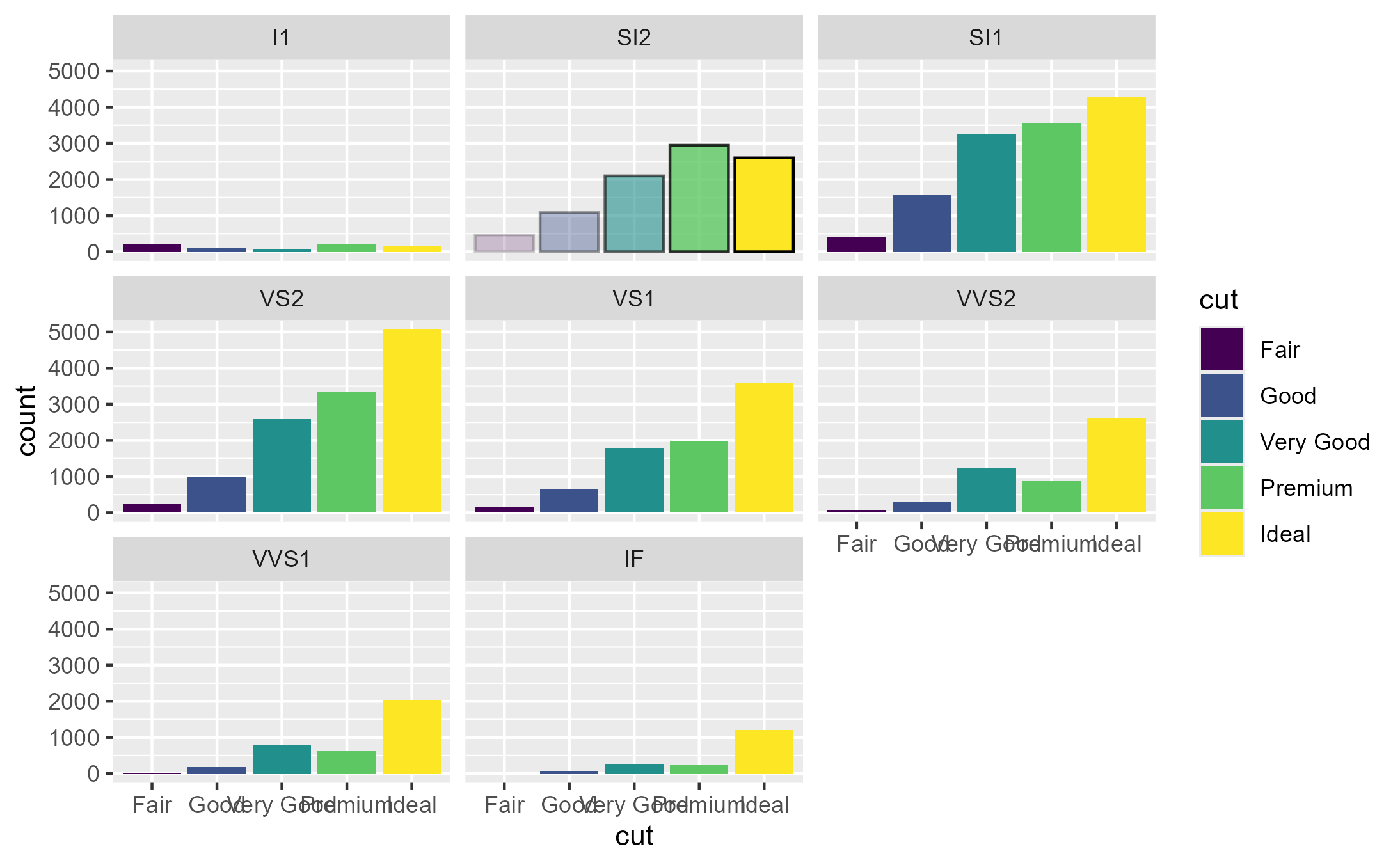Highjack a method's execution and make it return a user-supplied value

## Usage

ggtrace_highjack_return(
x,
method,
cond = 1L,
value = quote(returnValue()),
draw = TRUE
)

## Arguments

x

A ggplot object

method

A function or a ggproto method. The ggproto method may be specified using any of the following forms:

• ggproto$method • namespace::ggproto$method

• namespace:::ggproto$method cond When the return value should be replaced. Defaults to 1L. value What the method should return instead. Defaults to quote(returnValue()). draw Whether to draw the modified graphical output from evaluating x. Defaults to TRUE. ## Value A gtable object with class <ggtrace_highjacked> ## Tracing context When quoted expressions are passed to the cond or value argument of workflow functions they are evaluated in a special environment which we call the "tracing context". The tracing context is "data-masked" (see rlang::eval_tidy()), and exposes an internal variable called ._counter_ which increments every time a function/method has been called by the ggplot object supplied to the x argument of workflow functions. For example, cond = quote(._counter_ == 1L) is evaluated as TRUE when the method is called for the first time. The cond argument also supports numeric shorthands like cond = 1L which evaluates to quote(._counter_ == 1L), and this is the default value of cond for all workflow functions that only return one value (e.g., ggtrace_capture_fn()). It is recommended to consult the output of ggtrace_inspect_n() and ggtrace_inspect_which() to construct expressions that condition on ._counter_. For highjack functions like ggtrace_highjack_return(), the value about to be returned by the function/method can be accessed with returnValue() in the value argument. By default, value is set to quote(returnValue()) which simply evaluates to the return value, but directly computing on returnValue() to derive a different return value for the function/method is also possible. ## Examples  set.seed(1116) library(ggplot2) library(dplyr) p1 <- ggplot(diamonds, aes(cut)) + geom_bar(aes(fill = cut)) + facet_wrap(~ clarity) p1# Highjack Stat$compute_panel at the first panel
# to return higher values for count
ggtrace_highjack_return(
x = p1, method = Stat$compute_panel, value = quote({ returnValue() %>% mutate(count = count * 100) }) )# Highjack Stat$compute_panel at the fourth panel
# to shuffle bars in the x-axis
ggtrace_highjack_return(
x = p1, method = Stat$compute_panel, cond = quote(data$PANEL == 4),
value = quote({
returnValue() %>%
mutate(x = sample(x))
})
)# Bars get a black outline and get darker from left-to-right, but only for second panel
ggtrace_highjack_return(
x = p1, method = GeomBar$draw_panel, cond = quote(data$PANEL == 2),
value = quote({
editGrob(returnValue(), gp = gpar(
col = "black", alpha = seq(0.2, 1, length.out = nrow(data)
)))
})
)